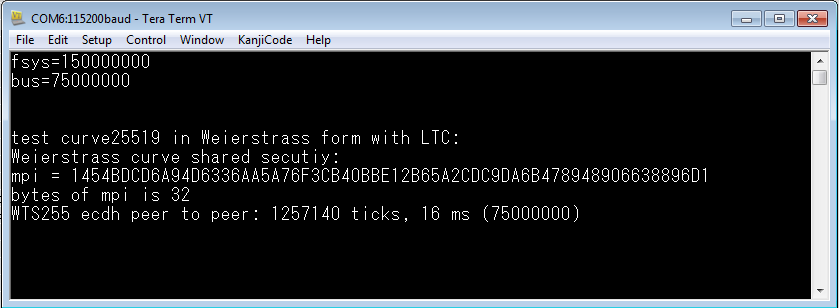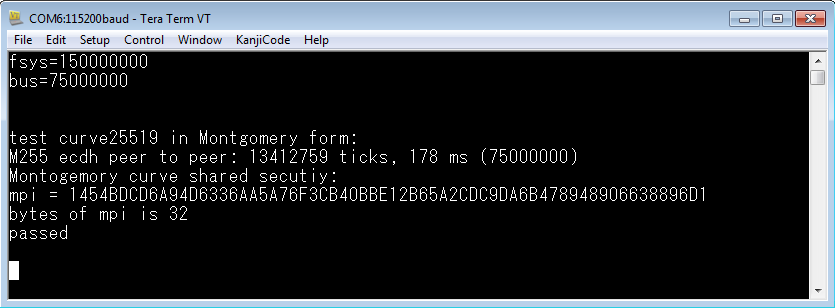# How to use the Kinets LTC ECC HW to accelerate Curve25519.

cancel
Showing results for
Did you mean:

# How to use the Kinets LTC ECC HW to accelerate Curve25519.

No ratings

## How to use the Kinets LTC ECC HW to accelerate Curve25519.

Curve22519 is a Montgomery elliptic-curve. Such as Apple HomeKit, most of network and IoT software use it

in Diffie-Hellman algorithm for key exchanging.

On the Security Kinets MCU chip,if we use just the software algorithm (base on mbedTLS), Curve25519 will spend

180ms for calculation of the shared security.

It is faster than other 256bit elliptic-curve with software algorithm, Because of the shared security

calculation will take more than 1200ms with a Weierstrass’s BP256R1curve when use software algorithm.

With LTC ECC HW acceleration, it take only 16ms to calculate the shared security on 256bit elliptic-curve.

Whatever you do, the speed of hardware acceleration always faster than the software algorithm.

Now that we should also want to use the LTC to accelerate the Curve22519. The LTC, however, only
supported Weierstrass form curve, but Curve22519 is a Montgomery curve…

Although, we can't use LTC in Curve22519 directly, we can use it by mapping it to a Weierstrass form
to use it.  As below, we gave parameters of these curves, transform formulas, example code and test result

to show how and why to do it.

1. Curve parameter:

Cuvre22519 in Montgomery form:

Y^2 = X^3 + A*X^2 + X

Fp = 0x7fffffffffffffffffffffffffffffffffffffffffffffffffffffffffffffed

A= 486662

Gx = 9

Gy = 0x20ae19a1b8a086b4e01edd2c7748d14c923d4d7e6d7c61b229e9c5a27eced3d9

Order of G point  =  0x1000000000000000000000000000000014def9dea2f79cd65812631a5cf5d3ed

Cuvre22519 in Weierstrass form :

Y^2 = X^3 + a*X + b

Fp = 0x7fffffffffffffffffffffffffffffffffffffffffffffffffffffffffffffed

a  =  0x2aaaaaaaaaaaaaaaaaaaaaaaaaaaaaaaaaaaaaaaaaaaaaaaaaaaaa984914a144L

b  =  0x7b425ed097b425ed097b425ed097b425ed097b425ed097b4260b5e9c7710c864L

Gy = 0x20ae19a1b8a086b4e01edd2c7748d14c923d4d7e6d7c61b229e9c5a27eced3d9

Order of G point  =  0x1000000000000000000000000000000014def9dea2f79cd65812631a5cf5d3ed

2. Calculation formula:

x_w –  x-coordinate value in  Weierstrass form

y_w –  y-coordinate value in  Weierstrass form

x_m - x-coordinate
value in  Montgomory form

y_m -  we don’t care y-coordinate value in  Weierstrass mode

a_m – a coefficient
of Montgomery equation (   Y^2 = X^3 + a_m * X^2 + X)

a_w – a coefficient
of Weierstrass equation (   Y^2 = X^3 + a*X + b )

b_w – a coefficient
of Weierstrass equation (   Y^2 = X^3 + a*X + b )

a)  x_w = (x_m + a_m/3)  %  p

b)  y_w ^2 = x_w ^ 3 + a_w*x_w + b_w

c)   x_m = (x_w - a_m/3) % p

You could reference these document as below:

https://en.wikipedia.org/wiki/Curve25519

https://en.wikipedia.org/wiki/Montgomery_curve

3. example code:

``````// public and private at Montgomery end
#define M255_Qx     "0x3BA5048381744348D84E754B9944ABE080B37F7D4158DCE60CD79F66B98AB89E"
// public and private at Weierstrass end
#define WTS255_d    "0x09CC5CCF43C656C1309EE5A3491D5A8361607CEEB0C9B2B31A575E0FEF2B8835"
#define WTS255_Qx   "0x3F4BDE110EE7AF71EF428D1018D188E35BAFB019F34F84E6465C5194B363DC2D"
#define WTS255_Qy   "0x7540577CE6F920354E2A9D38CE88847D7447E66FA4D188AC75CB63C17210B718"
#define WTS255_Qx_TO_M255_Qx     "0x14A13366643D04C74497E2656E26DE38B105056F48A4DA3B9BB1A6EA08B6B7DC"
int ecdh_wts_curve_end( )
{
unsigned int ticks;
int ret = 0;
size_t blen = 0, blen_peer = 0;
ecdh_context ecdh;
ecdh_context ecdh_peer;   // to_wts255
ecdh_context ecdh_peer_m255;
mpi R;
mpi_init(&R);
ecdh_init( &ecdh);
ecdh_init( &ecdh_peer);
ecdh_init( &ecdh_peer_m255);
MPI_CHK(ecp_use_known_dp( &ecdh.grp, ECP_DP_WTS25519 ));
MPI_CHK(ecp_use_known_dp( &ecdh_peer.grp, ECP_DP_WTS25519 ));
MPI_CHK(ecp_use_known_dp( &ecdh_peer_m255.grp, ECP_DP_M255 ));
blen = set_hash_buff(/*TEST_ECP_GRP_ID*/ECP_DP_WTS25519, &secret_buf, ecp_name);
if(blen == 0) {
ret = -1;
goto cleanup;
}
mpi_lset(&ecdh.Q.Z, 1);
mpi_init(&ecdh_peer_m255.Q.Y);
mpi_lset(&ecdh_peer_m255.Q.Z, 1);
// map M255 point to WTS255 point
my_timer_start();

mpi_mod_mpi(&ecdh_peer.Q.X, &ecdh_peer.Q.X, &ecdh_peer_m255.grp.P);

mpi_lset(&R, 3);
mpi_exp_mod (&ecdh_peer_m255.Q.Y , &ecdh_peer.Q.X, &R, &ecdh_peer_m255.grp.P, NULL);
mpi_mul_mpi(&R, &ecdh_peer.grp.A, &ecdh_peer.Q.X);
mpi_mod_mpi(&R, &R, &ecdh_peer.grp.P);

mpi_mod_mpi(&ecdh_peer_m255.Q.Y, &ecdh_peer_m255.Q.Y, &ecdh_peer.grp.P);
mpi_mod_sqrt(&ecdh_peer.Q.Y, &ecdh_peer_m255.Q.Y, &ecdh_peer_m255.grp.P);
// z = 1
mpi_lset(&ecdh_peer.Q.Z, 1);

MPI_CHK(ecp_copy(&ecdh.Qp,  &ecdh_peer.Q));
MPI_CHK(ecdh_calc_secret_wts2mont( &ecdh, &blen, secret_buf, blen, myrand, NULL));

mpi_sub_mpi(&ecdh_peer_m255.Q.X, &ecdh.Q.X, &R);
mpi_mod_mpi(&ecdh_peer_m255.Q.X, &ecdh_peer_m255.Q.X, &ecdh_peer_m255.grp.P);
ticks = my_timer_stop();
// print out message
mpi_printf_string( &ecdh.z, 16);
polarssl_printf("%s ecdh peer to peer: %lu ticks, %d ms (%d) \n", ecp_name , ticks, ticks / (CLOCK_SYS_GetPitFreq(0) / 1000),CLOCK_SYS_GetPitFreq(0) );

cleanup:
if( ret !=0 )
polarssl_printf( "%s test Unexpected error, return code = %08X\n", ecp_name, ret );
mpi_free(&R);
ecdh_free( &ecdh);
ecdh_free( &ecdh_peer);
ecdh_free( &ecdh_peer_m255);

return( 0 );

}

int ecdh_mont_curve_end( )
{
int verbose = 1;
unsigned int ticks;
int ret = 0;
size_t blen = 0, blen_peer = 0;
ecdh_context ecdh;
ecp_point Q_peer;          // peer public point
ecdh_init( &ecdh);
ecp_point_init( &Q_peer);
MPI_CHK(ecp_use_known_dp( &ecdh.grp, ECP_DP_M255 ));
blen_peer = set_hash_buff(ECP_DP_M255, &secret_buf_peer, ecp_name);
if(blen_peer == 0) {
ret = -1;
goto cleanup;
}

mpi_init(&ecdh.Q.Y);   // don't care Y, only init it
mpi_lset(&ecdh.Q.Z, 1);
mpi_init(&Q_peer.Y);
mpi_lset(&Q_peer.Z, 1);

MPI_CHK(ecp_copy(&ecdh.Qp,  &Q_peer));
my_timer_start();

MPI_CHK(ecdh_calc_secret( &ecdh, &blen_peer, secret_buf_peer, blen_peer, myrand, NULL));
ticks = my_timer_stop();
polarssl_printf("%s ecdh peer to peer: %lu ticks, %d ms (%d) \n", ecp_name , ticks, ticks / (CLOCK_SYS_GetPitFreq(0) / 1000),CLOCK_SYS_GetPitFreq(0) );
mpi_printf_string( &ecdh.z, 16);

cleanup:
if( ret !=0 && verbose != 0 )
polarssl_printf( "%s test Unexpected error, return code = %08X\n", ecp_name, ret );
ecdh_free( &ecdh);
ecp_point_free( &Q_peer);
if( verbose != 0 )

return( 0 );
}

‍‍‍‍‍‍‍‍‍‍‍‍‍‍‍‍‍‍‍‍‍‍‍‍‍‍‍‍‍‍‍‍‍‍‍‍‍‍‍‍‍‍‍‍‍‍‍‍‍‍‍‍‍‍‍‍‍‍‍‍‍‍‍‍‍‍‍‍‍‍‍‍‍‍‍‍‍‍‍‍‍‍‍‍‍‍‍‍‍‍‍‍‍‍‍‍‍‍‍‍‍‍‍‍‍‍‍‍‍‍‍‍‍‍‍‍‍‍‍‍‍‍‍‍‍‍‍‍‍‍‍‍‍‍‍‍‍‍‍‍‍‍‍‍‍‍‍‍‍‍‍‍‍‍‍‍‍‍‍‍‍‍‍‍‍‍‍‍‍‍‍‍‍‍‍‍‍‍‍‍‍‍‍‍‍‍‍‍‍‍‍‍‍‍‍‍‍‍‍‍‍‍‍‍‍‍‍‍‍‍‍‍‍‍‍‍‍‍‍‍‍‍‍‍‍‍‍‍‍‍‍‍‍‍‍‍‍‍‍‍‍‍‍‍‍‍‍‍‍‍‍‍‍‍‍‍‍‍‍‍‍‍‍‍‍‍‍‍‍‍‍‍‍‍‍‍‍‍‍‍‍‍‍‍‍‍‍‍‍‍‍‍‍‍‍‍‍‍‍‍‍‍‍‍‍‍‍‍‍‍‍‍‍‍‍‍‍‍‍‍‍‍‍‍‍‍‍‍‍‍‍‍‍‍‍‍‍‍‍‍‍‍‍‍‍‍‍‍‍‍‍‍‍‍‍‍‍‍‍‍‍‍‍‍‍‍‍‍‍‍‍‍‍‍‍‍‍‍‍‍‍‍‍‍‍‍‍‍‍‍‍‍‍‍‍‍‍‍‍‍‍‍‍‍‍‍‍‍‍‍‍‍‍‍‍‍‍‍‍‍‍‍‍‍‍‍‍‍‍‍‍‍‍‍‍‍‍‍‍‍‍‍‍‍‍‍‍‍‍‍‍‍‍‍‍‍‍‍‍‍‍‍‍‍‍‍‍‍‍‍‍‍‍‍‍‍‍‍‍‍‍‍‍‍‍‍‍‍‍‍‍‍‍‍‍‍‍‍‍‍‍‍‍‍‍‍‍‍‍‍‍‍‍‍‍‍‍‍‍‍‍‍‍‍‍‍‍‍‍‍‍‍‍‍‍‍‍‍‍‍‍‍‍‍‍‍‍‍‍‍‍‍‍‍‍‍‍‍‍‍‍‍‍‍‍‍‍‍‍‍‍‍‍‍‍‍‍‍‍‍‍‍‍‍‍‍‍‍‍‍‍‍‍‍‍‍‍‍‍‍‍‍‍‍‍‍‍‍‍‍‍‍‍‍‍‍‍‍‍‍‍‍‍‍‍‍‍‍‍‍‍‍‍‍‍‍‍‍‍‍‍‍‍‍‍‍‍‍‍‍‍‍‍‍‍‍‍‍‍‍‍‍‍‍‍‍‍‍‍‍‍‍‍‍‍‍‍‍‍‍‍‍‍‍‍‍‍‍‍‍‍‍‍‍‍‍‍‍‍‍‍‍‍‍‍‍‍‍‍‍‍‍‍‍‍‍‍‍‍‍‍‍‍‍‍‍‍‍‍‍‍‍‍‍‍‍‍‍‍‍‍‍‍‍‍‍‍‍‍‍‍‍‍‍‍‍‍‍‍‍‍‍‍‍‍‍‍‍‍‍‍‍‍``````

4. Test result:

1. Test result of curv25519 in  Weierstrass form with LTC:2. Test result of curve25519 in Montgomery form with software algorithm:We could see that the shared security both in Weierstrass form with LTC and Montgomery form are “0x1454BDCD6A94D6336AA5A76F3CB40BBE12B65A2CDC9DA6B478948906638896D1”.
But the calculation speed with LTC was ten times faster than other one.

Labels (1)
• ### Kinetis K Series MCUs

Version history
Revision #:
2 of 2
Last update:
‎09-10-2020 01:48 AM
Updated by: